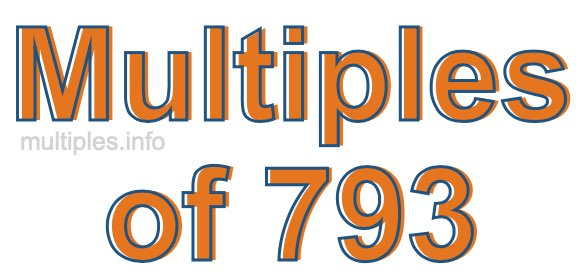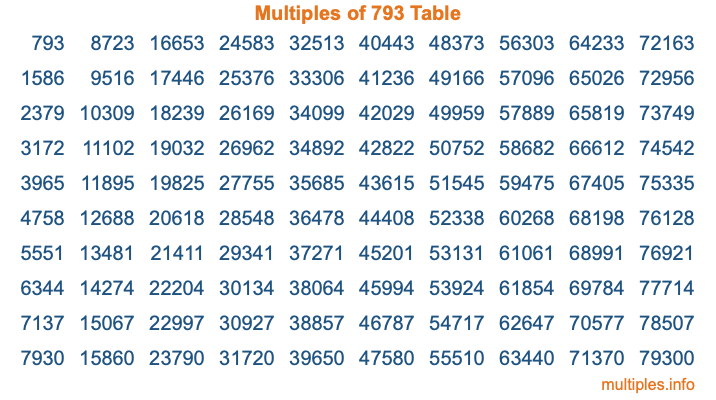Multiples of 793Welcome to the Multiples of 793 page. Here we will first teach you everything you will ever need to know about the multiples of 793, and then give you a study guide summary of everything we taught you to make sure you remember it all. Use this page to look up facts and learn information about the multiples of 793. This page will make you a multiples of seven hundred ninety-three expert!

Definition of Multiples of 793
Multiples of 793 are all the numbers that when divided by 793 equal an integer. Each of the multiples of 793 are called a multiple. A multiple of 793 is created by multiplying 793 by an integer.

Therefore, to create a list of multiples of 793, you start with 1 multiplied by 793, then 2 multiplied by 793, then 3 multiplied by 793, and so on for as long as you want. Thus, the list of the first five multiples of 793 is 793, 1586, 2379, 3172, and 3965. To see a larger list of multiples of 793, see the printable image of Multiples of 793 further down on this page. We also have a category where you can choose any nth multiple of 793.

Multiples of 793 Checker
The Multiples of 793 Checker below checks to see if any number of your choice is a multiple of 793. In other words, it checks to see if there is any number (integer) that when multiplied by 793 will equal your number. To do that, we divide your number by 793. If the the quotient is an integer, then your number is a multiple of 793.

Is  a multiple of 793?

Least Common Multiple of 793 and ...
A Least Common Multiple (LCM) is the lowest multiple that two or more numbers have in common. This is also called the smallest common multiple or lowest common multiple and is useful to know when you are adding our subtracting fractions. Enter one or more numbers below (793 is already entered) to find the LCM.

Check out our LCM Calculator if you need more details about the Least Common Multiple or if you need the LCM for different numbers for adding and subtraction fractions.

nth Multiple of 793
As we stated above, 793 is the first multiple of 793, 1586 is the second multiple of 793, 2379 is the third multiple of 793, and so on. Enter a number below to find the nth multiple of 793.

th multiple of 793

Multiples of 793 vs Factors of 793
793 is a multiple of 793 and a factor of 793, but that is where the similarities end. All postive multiples of 793 are 793 or greater than 793. All positive factors of 793 are 793 or less than 793.

Below is the beginning list of multiples of 793 and the factors of 793 so you can compare:

Multiples of 793: 793, 1586, 2379, 3172, 3965, etc.

Factors of 793: 1, 13, 61, 793

As you can see, the multiples of 793 are all the numbers that you can divide by 793 to get a whole number. The factors of 793, on the other hand, are all the whole numbers that you can multiply by another whole number to get 793.

It's also interesting to note that if a number (x) is a factor of 793, then 793 will also be a multiple of that number (x).

Multiples of 793 vs Divisors of 793
The divisors of 793 are all the integers that 793 can be divided by evenly. Below is a list of the divisors of 793.

Divisors of 793: 1, 13, 61, 793

The interesting thing to note here is that if you take any multiple of 793 and divide it by a divisor of 793, you will see that the quotient is an integer.

Multiples of 793 Table
Below is an image of the first 100 multiples of 793 in a table. The table is in chronological order, column by column. The first column has the first ten multiples of 793, the second column has the next ten multiples of 793, and so on.The Multiples of 793 Table is also referred to as the 793 Times Table or Times Table of 793. You are welcome to print out our table for your studies.

Negative Multiples of 793
Although not often discussed or needed in math, it is worth mentioning that you can make a list of negative multiples of 793 by multiplying 793 by -1, then by -2, then by -3, and so on, to get the following list of negative multiples of 793:

-793, -1586, -2379, -3172, -3965, etc.

Multiples of 793 Summary
Below is a summary of important Multiples of 793 facts that we have discussed on this page. To retain the knowledge on this page, we recommend that you read through the summary and explain to yourself or a study partner why they hold true.

There are an infinite number of multiples of 793.

A multiple of 793 divided by 793 will equal a whole number.

793 divided by a factor of 793 equals a divisor of 793.

The nth multiple of 793 is n times 793.

The largest factor of 793 is equal to the first positive multiple of 793.

793 is a multiple of every factor of 793.

793 is a multiple of 793.

A multiple of 793 divided by a divisor of 793 equals an integer.

793 divided by a divisor of 793 equals a factor of 793.

Any integer times 793 will equal a multiple of 793.

Multiples of a Number
Here you can get the multiples of another number, all with the same attention to detail as we did for multiples of 793 on this page.

Multiples of
Multiples of 794
Did you find our page about multiples of seven hundred ninety-three educational? Do you want more knowledge? Check out the multiples of the next number on our list!